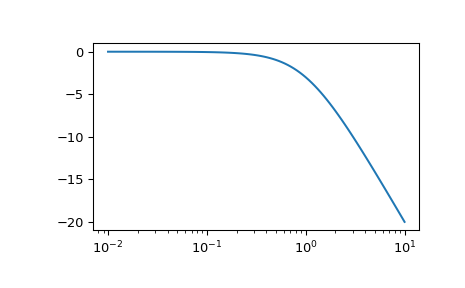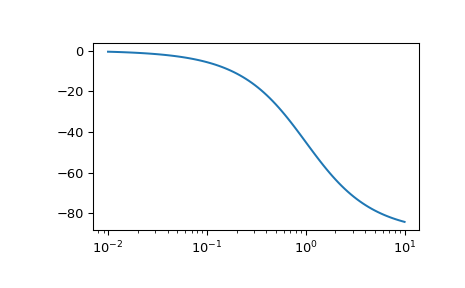# scipy.signal.bode¶

scipy.signal.bode(system, w=None, n=100)[source]

Calculate Bode magnitude and phase data of a continuous-time system.

Parameters
systeman instance of the LTI class or a tuple describing the system.

The following gives the number of elements in the tuple and the interpretation:

• 1 (instance of `lti`)

• 2 (num, den)

• 3 (zeros, poles, gain)

• 4 (A, B, C, D)

warray_like, optional

Array of frequencies (in rad/s). Magnitude and phase data is calculated for every value in this array. If not given a reasonable set will be calculated.

nint, optional

Number of frequency points to compute if w is not given. The n frequencies are logarithmically spaced in an interval chosen to include the influence of the poles and zeros of the system.

Returns
w1D ndarray

mag1D ndarray

Magnitude array [dB]

phase1D ndarray

Phase array [deg]

Notes

If (num, den) is passed in for `system`, coefficients for both the numerator and denominator should be specified in descending exponent order (e.g. `s^2 + 3s + 5` would be represented as `[1, 3, 5]`).

New in version 0.11.0.

Examples

```>>> from scipy import signal
>>> import matplotlib.pyplot as plt
```
```>>> sys = signal.TransferFunction(, [1, 1])
>>> w, mag, phase = signal.bode(sys)
```
```>>> plt.figure()
>>> plt.semilogx(w, mag)    # Bode magnitude plot
>>> plt.figure()
>>> plt.semilogx(w, phase)  # Bode phase plot
>>> plt.show()
```